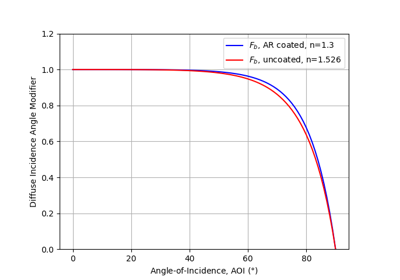# pvlib.iam.marion_diffuse#

pvlib.iam.marion_diffuse(model, surface_tilt, **kwargs)[source]#

Determine diffuse irradiance incidence angle modifiers using Marion’s method of integrating over solid angle.

Parameters
• model (str) – The IAM function to evaluate across solid angle. Must be one of ‘ashrae’, ‘physical’, ‘martin_ruiz’, ‘sapm’, ‘schlick’.

• surface_tilt (numeric) – Surface tilt angles in decimal degrees. The tilt angle is defined as degrees from horizontal (e.g. surface facing up = 0, surface facing horizon = 90).

• **kwargs – Extra parameters passed to the IAM function.

Returns

iam (dict) –

IAM values for each type of diffuse irradiance:

• ’sky’: radiation from the sky dome (zenith <= 90)

• ’horizon’: radiation from the region of the sky near the horizon (89.5 <= zenith <= 90)

• ’ground’: radiation reflected from the ground (zenith >= 90)

See 1 for a detailed description of each class.

References

1

B. Marion “Numerical method for angle-of-incidence correction factors for diffuse radiation incident photovoltaic modules”, Solar Energy, Volume 147, Pages 344-348. 2017. DOI: 10.1016/j.solener.2017.03.027

Examples

```>>> marion_diffuse('physical', surface_tilt=20)
{'sky': 0.9539178294437575,
'horizon': 0.7652650139134007,
'ground': 0.6387140117795903}
```
```>>> marion_diffuse('ashrae', [20, 30], b=0.04)
{'sky': array([0.96748999, 0.96938408]),
'horizon': array([0.86478428, 0.91825792]),
'ground': array([0.77004435, 0.8522436 ])}
```

## Examples using `pvlib.iam.marion_diffuse`#Diffuse IAM Calculation

Diffuse IAM Calculation